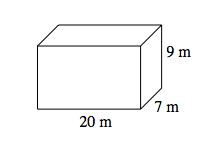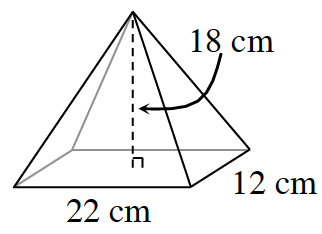### Home > ACC7 > Chapter 10 Unit 11 > Lesson CC3: 10.1.4 > Problem10-57

10-57.

Find the volume of each shape below.

1. Rectangle-based prismThe volume of a rectangle-based prism is:
$V=\left(\text{base area}\right)\left(\text{height}\right)$

Substitute the values given.
$V=\left[\left(20\ \text{m}\right)\left(7\ \text{m}\right)\right]\left[9\ \text{m}\right]$

Evaluate.
$V=\left[140\ \text{m}^2\right]\left[9\ \text{m}\right]$

$1260\ \text{m}^3$

1. Rectangle-based pyramidFrom the math notes, the volume of a pyramid is $\frac{1}{3}$(Base Area)(height). Then follow the steps from part (a).